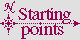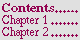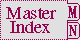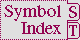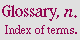#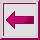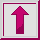Function =, /=, <, >, <=, >=

Syntax:

= &rest numbers+ => generalized-boolean

/= &rest numbers+ => generalized-boolean

< &rest numbers+ => generalized-boolean

> &rest numbers+ => generalized-boolean

<= &rest numbers+ => generalized-boolean

>= &rest numbers+ => generalized-boolean

Arguments and Values:

number---for <, >, <=, >=: a real; for =, /=: a number.

generalized-boolean---a generalized boolean.

Description:

=, /=, <, >, <=, and >= perform arithmetic comparisons on their arguments as follows:

=

The value of = is true if all numbers are the same in value; otherwise it is false. Two complexes are considered equal by = if their real and imaginary parts are equal according to =.

/=

The value of /= is true if no two numbers are the same in value; otherwise it is false.

<

The value of < is true if the numbers are in monotonically increasing order; otherwise it is false.

>

The value of > is true if the numbers are in monotonically decreasing order; otherwise it is false.

<=

The value of <= is true if the numbers are in monotonically nondecreasing order; otherwise it is false.

>=

The value of >= is true if the numbers are in monotonically nonincreasing order; otherwise it is false.

=, /=, <, >, <=, and >= perform necessary type conversions.

Examples:

The uses of these functions are illustrated in the next figure.

```(= 3 3) is true.              (/= 3 3) is false.
(= 3 5) is false.             (/= 3 5) is true.
(= 3 3 3 3) is true.          (/= 3 3 3 3) is false.
(= 3 3 5 3) is false.         (/= 3 3 5 3) is false.
(= 3 6 5 2) is false.         (/= 3 6 5 2) is true.
(= 3 2 3) is false.           (/= 3 2 3) is false.
(< 3 5) is true.              (<= 3 5) is true.
(< 3 -5) is false.            (<= 3 -5) is false.
(< 3 3) is false.             (<= 3 3) is true.
(< 0 3 4 6 7) is true.        (<= 0 3 4 6 7) is true.
(< 0 3 4 4 6) is false.       (<= 0 3 4 4 6) is true.
(> 4 3) is true.              (>= 4 3) is true.
(> 4 3 2 1 0) is true.        (>= 4 3 2 1 0) is true.
(> 4 3 3 2 0) is false.       (>= 4 3 3 2 0) is true.
(> 4 3 1 2 0) is false.       (>= 4 3 1 2 0) is false.
(= 3) is true.                (/= 3) is true.
(< 3) is true.                (<= 3) is true.
(= 3.0 #c(3.0 0.0)) is true.  (/= 3.0 #c(3.0 1.0)) is true.
(= 3 3.0) is true.            (= 3.0s0 3.0d0) is true.
(= 0.0 -0.0) is true.         (= 5/2 2.5) is true.
(> 0.0 -0.0) is false.        (= 0 -0.0) is true.
(<= 0 x 9) is true if x is between 0 and 9, inclusive
(< 0.0 x 1.0) is true if x is between 0.0 and 1.0, exclusive
(< -1 j (length v)) is true if j is a valid array index for a vector v
```

Figure 12-13. Uses of /=, =, <, >, <=, and >=

Affected By: None.

Exceptional Situations:

Might signal type-error if some argument is not a real. Might signal arithmetic-error if otherwise unable to fulfill its contract.